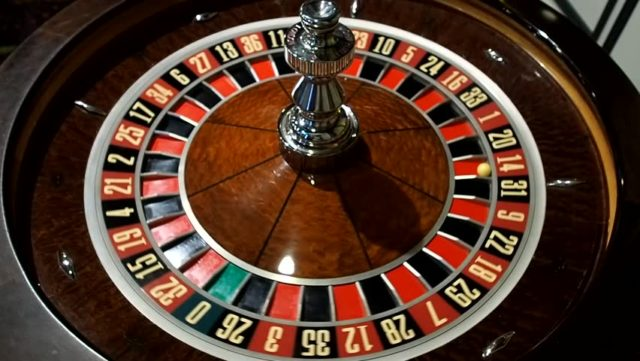The roulette wheel selection is also known as the fitness proportionate selection. It is an operator that is mostly used for parent selection by using genetic algorithms to find the best match.

To understand this function, let’s consider an actual roulette wheel. Say this circular wheel is divided into n pies (like a pie chart), where n represents the number of potential parents in the community.

Since the selection process considers the fitness of these individuals, this means that each individual occupies a space on the wheel which is proportional to their fitness value. Individuals with higher fitness stats have a higher chance of mating and passing on those traits to their offspring. If this goes on, eventually, better and stronger individuals will be born over time. An evolution of some sort.

### How Does it Work?

The roulette wheel selection is an algorithm that is used to select an item that corresponds to its probability or odds. For example, suppose we have five items on a wheel, numbered (0, 1, 2, 3, 4).

Again, suppose we want to set the probabilities of each of those items to (0.2, 0.5, 0.3, 0.4, 0.1) respectively. This means that we want item  to have a selection probability (sp) = 0.2, item  to have a selection probably = 0.5, item  to have sp = 0.3, item  to have sp = 0.4, and item  to have sp = 0.1.

To do this, we would have to calculate the cumulative probabilities.

(0.20, 0.70, 1.00, 1.40, 1.50)

After this, we can generate a random probability value P between the lowest number, 0.0 and the final cumulative probability, 1.50. If P falls between (0.0, 0.20) we select item . If P falls between (0.20, 0.70) we select item . If P falls between (0.70, 1.00) we select item . If P falls between (1.00, 1.40) we select item . If P falls between (1.40, 1.50) we select item .### The Fixed Point Approach

Still using the roulette wheel as our basis, and the “item” as potential parents (individuals), we can try the fixed point approach. With the circular wheel already divided, we can choose any part on the wheel circumference as our fixed point.

Once this fixed point has been marked, the wheel is rotated. The section of the wheel which stops directly in front of the fixed point is chosen as the parent. This process is repeated to select the second parent.

Since the parameter for choosing a parent is fitness, a fitter individual would occupy a greater region on the wheel and therefore have a higher chance of stopping in front of the fixed point when the wheel stops rotating.

To implement this, we use the following steps:

• Calculate the sum of the fitness values, S.
• Generate a random number between 0 and S.
• From the top of the population, start adding the fitness values to the partial sum P until P becomes less than S.
• The point (individual) at which the value of P exceeds S, is the chosen parent.

### The Game Itself

This algorithm can be used to calculate the odds of an actual Roulette game by replacing the “items” or “individuals” with roulette pockets. Here, the sizes of the different pockets are proportionate to the probability of them getting picked. Therefore, a player with a greater number of pockets (wider area) will be less likely to be eliminated and more likely to win.

### Summary

• The winner will be selected according to their pockets (i.e. the pocket is the selection parameter)
• The more pockets a player has, the greater their chances of being selected.